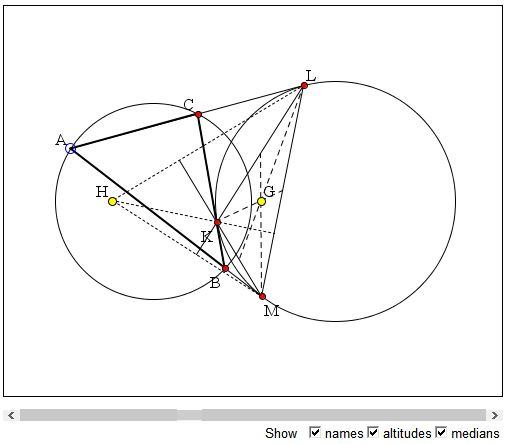# Extouch Triangle in Poncelet Porism What is this about? A Mathematical Droodle

### If you are reading this, your browser is not set to run Java applets. Try IE11 or Safari and declare the site https:///www.cut-the-knot.org as trusted in the Java setup.What if applet does not run?

ExplanationThe applet attempts to illustrate a property of the extouch triangles involved in the Poncelet porism: given two circles, O(R) and I(r), let ABC be a generic triangle inscribed into O(R) for which I(r) serves as an excircle. If d, the distance between the centers of the circles satisfies 1/(d - R) - 1/(d + R) = 1/r, there is a continuum of such triangles. Construct, for each such triangle, the corresponding intouch triangle KLM. And, for the latter, find the orthocenter H and the (bary)center G. Surprisingly, for the given two circles, neither depends on the selection of triangle ABC. Since the incenter of ΔABC which serves as the circumcenter of ΔKLM is also fixed, we may conclude that the entire Euler line is fixed point-by-point implying, for example, that the 9-point center of ΔKLM is also independent of the position of ΔABC.

### If you are reading this, your browser is not set to run Java applets. Try IE11 or Safari and declare the site https:///www.cut-the-knot.org as trusted in the Java setup.What if applet does not run?

... to be continued ...

### References

1. L. Emelyanov and T. Emelyanova, Euler’s Formula and Poncelet’s Porism, Forum Geometricorum, Volume 1 (2001) 137–140
2. W. Gallatly, The Modern Geometry of the Triangle, Scholarly Publishing Office, University of Michigan Library (December 20, 2005)#### Poncelet Porism